# DK Goel Solutions Class 11 Accountancy Chapter 21 Financial Statement

Read DK Goel Class 11 Accountancy Solutions for Chapter 21 Financial Statement below. These DK Goel Accountancy Class 11 solutions have been prepared based on the latest book for DK Goel Class 11 for the current academic year by expert accounts teachers at studiestoday.com. These DK Goel Class 11 Solutions help commerce students in class 11 understand accountancy and build a strong base in accounts. Students in Class 11 who study accountancy and use the DK Goel Accountancy book to understand concepts of Chapter 21 Financial Statement should understand the concepts and solve practice questions and exercises given at the end of the chapter. We have provided solutions for all questions and have also provided short notes for each problem. This will help Class 11 DK Goel Accountancy students to understand the questions properly. Refer to the solutions provided below prepared by CBSE NCERT teachers

## Chapter 21 Financial Statement DK Goel Class 11 Solutions

Class 11 Accountancy students should read the following DK Goel Solutions for Class 11 Chapter 21 Financial Statement in Standard 11. All solutions provided below can be downloaded in Pdf and are available for free. This DK Goel Book for Grade 11 Accountancy will be very useful for exams and help you to score good marks in Class 11 accountancy examinations. On our website www.studiestoday.com, we have provided solutions for all chapters given in the DK Goel Accountancy Book for Class 11.

### DK Goel Solutions Chapter 21 Financial Statement Class 11 Accountancy

Question 1. What is the used of financial statement for employees and trade union?

Solution  1: On the basis of financial statement they can judge as to how much bonus and increase in their wages is possible from the profits of the enterprise.

Question 2. What is the use of financial statement for potential investors?

Solution  2: They can assess the short-term and long –term financial soundness and earning capacity of the business with the help of financial statement. They can also study the trend of sales, trend of profit, shortcomings and the prospects of future growth of the enterprise.

Question 3. What is meant by operating profit?

Solution  3: Operating profit is the profit earned through normal operating activities of the business. It is arrived at by deducting the operating express from gross profit. Expenses which are related to the main or normal activities of the business are called operating expenses.

Question 4. What is meant by Indirect Expenses? Give two examples.

Solution  4: Indirect expenses are those expenses which are incurred and are not directly associated with the purchases of goods or manufacture of goods.

The two examples of indirect expenses are:

1. Office salaries.
2. Warehouse expenses.

Question 5. Give four points of distinction between Trading Account & Profit and Loss Account.

Solution  5: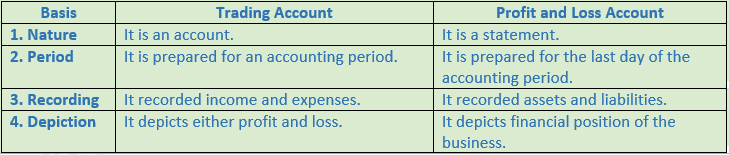Question 6. What purpose does a Balance Sheet serve?

Solution  6: A balance sheet is prepared to ascertain the financial position of an enterprise on a particular date. It shows liabilities on the left hand side and assets on right hand side.

Question 7. What is meant by “Grouping' and 'Marshalling' a Balance Sheet?

Solution  7: Grouping means showing the assets or liabilities of similar nature common heading and Marshalling means showing the assets and liabilities in a proper order.

Question 8. Write the various Assets in order of liquidity in a Balance Sheet.

Solution  8: According to this method an asset which is most easily convertible into cash such as cash in hand is written first and then will follow those assets which are comparatively less easily convertible so that the least liquid asset such as goodwill is shown last.

Question 9. When assets are listed in order of their liquidity in a Balance Sheet, which should be listed first — Buildings or Cash in hand?

Solution  9: An asset which is most easily convertible into cash such as cash in hand is written first and then will follow those assets which are comparatively less easily convertible so that the least liquid asset such as goodwill is shown last.

Question 10. Explain the Current Assets and Non-Current Assets.

Solution  10: Current Assets are those assets which are held for resale or for converting into cash. These are the assets which are likely to be realized within a period of one year or during the period of normal operating cycle. A business earns profit by selling these assets but not by keeping them as stock for a longer period. These assets are temporary in nature and may change from time to time. These are sometimes referred to as floating or circulating assets.

Non-Current Assets are those assets are which are acquired for continuous use and last for many years such as Land and Building, Plant and Machinery, Motor Vehicles, Furniture etc.

Question 11. Give two examples of each of Fixed Assets and Current Assets.

Solution  11: Example of Fixed Assets:-

1.) Land and Building

2.) Plant and Machinery

Example of Current Assets:-

1.) Cash

2.) Stock

Question 12. What do you mean by ‘Contingent Liabilities'? Give its two examples.

Solution  12: These are the liabilities which will become payable only on the happing of some specific event, otherwise not such as:-

(i) Liabilities for bill discounted

(ii) Liabilities in respect of a suit pending in a court of law

(iii) Liability in respect of a guarantee give for another person.

Question 13. Give any five points of difference between a Balance Sheet and a Trial Balance.

Solution  13: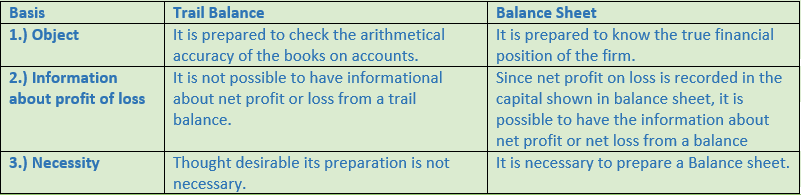Question 14. When liabilities are listed in order of liquidity in a Balance Sheet, which should be listed first — Capital or Creditors?

Solution  14: Those liabilities which are to be paid at the earliest will be written first. In other words, current liabilities are written first of all, then non-current or long-term liabilities and lastly the proprietor’s capital.

Numerical Questions

Question 1. Prepare a Trading Account from the following particulars for the year ended 31st March 2017:-Closing Stock is valued at Rs. 6,00,000.

Solution 1:Point of Knowledge:-

Trading Account is the account that reveals the gross profit or gross loss. It is credited with the amount of sales of goods and debited with the opening stock of goods along with the direct expenses related to the sales made. Trading Account is prepared to know gross profit or gross loss during the accounting period.

Question 2. (A) From the following information, prepare the Trading Account for the year ended 31st March, 2017:

Adjusted Purchases Rs. 15,00,000; Sales Rs. 21,40,000; Returns Inwards Rs. 40,000; Freight and Packing Rs. 15,000; Packing Expenses on Sales Rs. 20,000; Depreciation Rs. 36,000; Factory Expenses Rs. 60,000; Closing Stock Rs. 1,20,000.

Solution 2:  (A)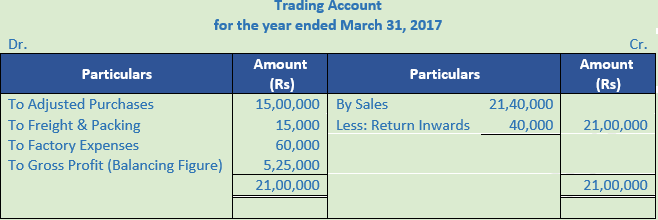Working Note:-

Calculation of Adjusted Purchases = Opening Stock + Net Purchases – Closing Stock

Point of Knowledge:-

Closing stock is not showing separately in trading account as it is already subtracted in adjusted purchases.

Question 2. (B) Calculate Gross Profit from the following information:Working Note:-

Calculation of Adjusted Purchases = Opening Stock + Net Purchases – Closing Stock

Point of Knowledge:-

Closing stock is not showing separately in trading account as it is already subtracted in adjusted purchases.

Question 3. (A) Calculate cost of goods sold from the following: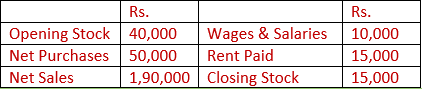Solution 3: (A) Calculation of Cost of Goods Sold:-

Cost of Goods Sold = Opening Stock + Purchases + Direct Expenses – Closing Stock

Cost of Goods Sold = 40,000 + 50,000 + 10,000 – 15,000

Cost of Goods Sold = Rs. 85,000

Question 3. (B) Ascertain cost of Goods Sold and Gross Profit from the following:Solution 3 (B):

Calculation of Gross Profit:-

Gross Profit = Net Sales – COGS (Cost of goods sold)

Gross Profit = Rs. 3,92,000 – Rs. 3,92,000

Gross Profit = Rs. 1,10,000

Calculation of Cost of Goods Sold:-

Cost of goods sold = Opening Stock + Purchases + Direct Expenses – Closing Stock

Cost of goods sold = Rs. 32,000 + Rs. 2,80,000 + Rs. 20,000 – Rs. 50,000

Cost of goods sold = Rs. 2,82,000

Calculation of Net Sales:-

Net Sales = Sales – Sales Return

Net Sales = Rs. 4,00,000 – Rs. 8,000

Net Sales = Rs. 3,92,000

Question 4. Calculate Gross Profit on the basis of the following information: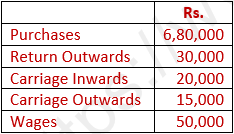¾ of the goods are sold for Rs. 6,00,000

Solution 4 :

Calculation of Gross Profit:-

Gross Profit = Net Sales – COGS (Cost of goods sold)

Gross Profit = Rs. 6,00,000 – Rs. 5,40,000

Gross Profit = Rs. 60,000

Calculation of Cost of Goods Sold:-

Cost of goods sold = Opening Stock + Purchases + Direct Expenses – Closing Stock

Cost of goods sold = Rs. 0 + Rs. 6,50,000 + Rs. 70,000 – Rs. 0

Cost of goods sold = Rs. 7,20,000

Cost of goods sold = ¾ × 7,20,000

Cost of goods sold = Rs. 5,40,000

Calculation of Net Purchases:-

Net Purchases = Purchases – Purchases Return

Net Purchases = Rs. 6,80,000 – Rs. 30,000

Net Purchases = Rs. 6,50,000

Calculation of Direct Expenses:-

Direct Expenses = Carriage Inwards + Wages

Direct Expenses = Rs. 20,000 + Rs. 50,000

Direct Expenses = Rs. 70,000

Question 5. (A) Calculate Closing Stock and Cost of Goods Sold:

Opening Stock Rs. 5,000; Sales Rs. 16,000; Carriage Inwards Rs. 1,000; Sales Returns Rs. 1,000; Gross Profit Rs. 6,000; Purchase Rs. 10,000; Purchase Returns Rs. 900.

Solution 5: (A)

Calculation of Closing Stock:-

Cost of goods sold = Opening Stock + Purchases + Direct Expenses – Closing Stock

Closing Stock = Opening Stock + Purchases + Direct Expenses – COGS

Closing Stock = Rs. 5,000 + Rs. 9,100 + Rs. 1,000 – Rs. 9,000

Closing Stock = Rs. 6,100

Calculation of Cost of Goods Sold:-

Cost of goods sold = Net Sales – Gross Profit

Cost of goods sold = Rs. 15,000 – Rs. 6,000

Cost of goods sold = Rs. 9,000

Calculation of Net Purchases:-

Net Purchases = Purchases - Purchases Return

Net Purchases = Rs. 10,000 - Rs. 900

Net Purchases = Rs. 9,100

Question 5. (B) Calculate Closing Stock from the following: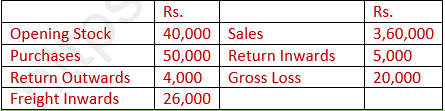Solution 5(B):

Calculation of Closing Stock:-

Cost of goods sold = Opening Stock + Purchases + Direct Expenses – Closing Stock

Closing Stock = Opening Stock + Purchases + Direct Expenses – COGS

Closing Stock = Rs. 38,000 + Rs. 3,36,000 + Rs. 26,000 – Rs. 3,75,000

Closing Stock = Rs. 25,000

Calculation of Cost of Goods Sold:-

Cost of goods sold = Net Sales + Gross Loss

Cost of goods sold = Rs. 3,60,000 – Rs. 5,000 + Rs. 20,000

Cost of goods sold = Rs. 3,75,000

Calculation of Net Purchases:-

Net Purchases = Purchases - Purchases Return

Net Purchases = Rs. 3,40,000 - Rs. 4,000

Net Purchases = Rs. 3,36,000

Question 6. (A) Calculate gross profit and cost of goods sold from the following information:

Net Sales Rs. 8,00,000

Gross Profit is 40% on Sales

Solution 6: (A) Net Sales = Rs. 8,00,000

Gross Profit = Sales × 40%

Gross Profit = Rs. 8,00,000 × 40%

Gross Profit = Rs. 3,20,000

Cost of Goods Sold = Sales – Gross Profit

Cost of Goods Sold = Rs. 8,00,000 – Rs. 3,20,000

Cost of Goods Sold = Rs. 4,80,000

Question 6. (B) Calculate gross profit and cost of goods sold from the following information: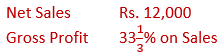Solution 6: (B)Cost of Goods Sold = Sales – Gross Profit

Cost of Goods Sold = Rs. 12,00,000 – Rs. 4,00,000

Cost of Goods Sold = Rs. 8,00,000

Question 7. Calculate the gross profit and cost of goods sold from the following information:

Net Sales Rs. 9,00,000

Gross Profit is 20% on cost.

Solution 7:

Net Sales = Rs. 9,00,000

Gross Profit = Sales × 20%

We know,Cost of Goods Sold = Sales – Gross Profit

Cost of Goods Sold = Rs. 9,00,000 – Rs. 1,50,000

Cost of Goods Sold = Rs. 7,50,000

Question 8. Ascertain the value of closing stock from the following: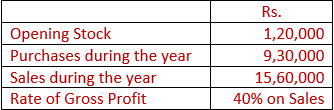Solution 8:

Calculation of Closing Stock:-

Cost of goods sold = Opening Stock + Purchases + Direct Expenses – Closing Stock

Closing Stock = Opening Stock + Purchases + Direct Expenses – COGS

Closing Stock = Rs. 1,20,000 + Rs. 9,30,000 + Rs. 0 – Rs. 9,36,000

Closing Stock = Rs. 10,50,000 – Rs. 9,36,000

Closing Stock = Rs. 1,14,000

Calculation of Cost of Goods Sold:-

Cost of Goods sold = Net Sales – Gross Profit

Cost of Goods sold = Rs. 15,60,000 – Rs. 6,24,000

Cost of Goods sold = Rs. 9,36,000

Calculation of Gross Profit:-

Gross Profit = 40% of Sales

Gross Profit = Rs. 15,60,000 × 40%

Gross Profit = Rs. 6,24,000

Question 9. Calculate closing stock from the following details: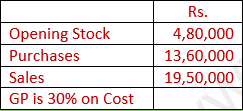Solution 9:

Calculation of Closing Stock:-

Cost of goods sold = Opening Stock + Purchases + Direct Expenses – Closing Stock

Closing Stock = Opening Stock + Purchases + Direct Expenses – COGS

Closing Stock = Rs. 4,80,000 + Rs. 13,60,000 + Rs. 0 – Rs. 15,00,000

Closing Stock = Rs. 18,40,000 – Rs. 15,00,000

Closing Stock = Rs. 3,40,000Calculation of Cost of Goods Sold:-

Cost of Goods Sold = Sales – Gross Profit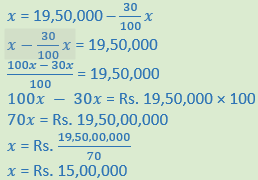Question 10. Calculate Net Sales and G.P. from the following:

Cost of Goods Sold          Rs. 4,50,000

G.P.                               25% on Sales

Solution 10 :         Gross Profit = 25% on sales

We know,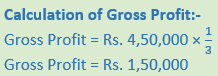Calculation of Sales:-

Cost of goods sold = Sales + Gross Profit

Sales = Cost of goods sold + Gross Profit

Sales = Rs. 4,50,000 + Rs. 1,50,000

Sales = Rs. 6,00,000

Question 11. Prepare Profit and Loss Account for the year ended 31st March, 2017 from the following particulars:-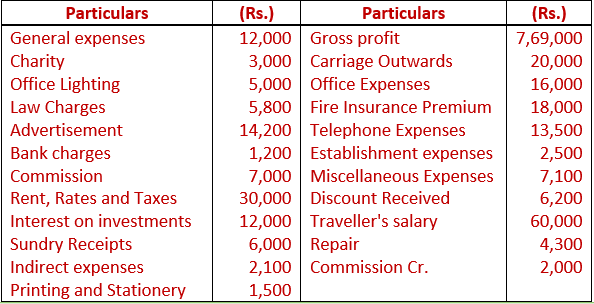Solution 11: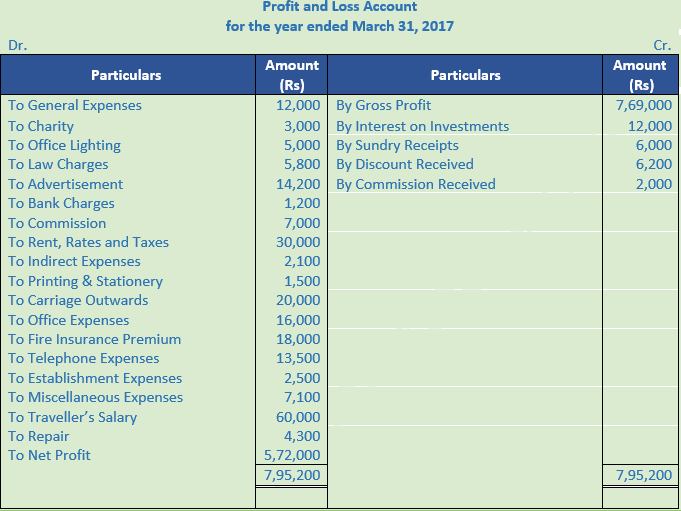Question 12. Calculate the amount of gross profit, operating profit and net profit on the basis of the following balances extracted from the books of M/s Rajiv & Sons for the year ended March 31, 2017.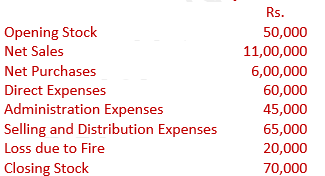Solution 12: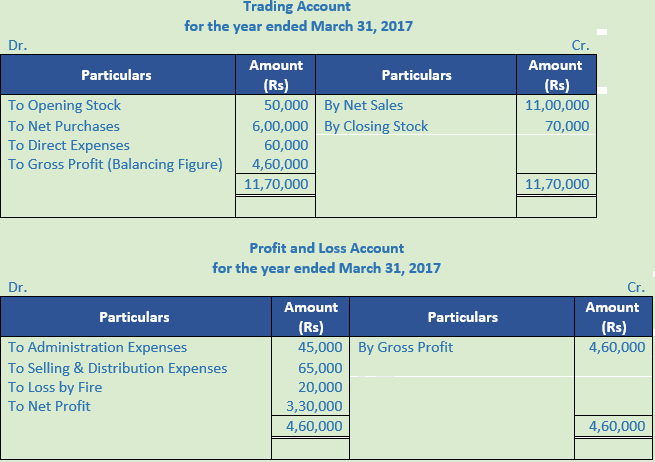Working Note:-

Calculation of Operating Profit:-

Operating Profit = Net Profit – Non-Operating Income + Non-Operating Expenses

Operating Profit = Rs. 3,30,000 – Rs. 0 + Rs. 20,000

Operating Profit = Rs. 3,50,000

Point of Knowledge:-

Trading Account is the account that reveals the gross profit or gross loss. It is credited with the amount of sales of goods and debited with the opening stock of goods along with the direct expenses related to the sales made. Trading Account is prepared to know gross profit or gross loss during the accounting period.

Question 13. Calculate operating profit from the following: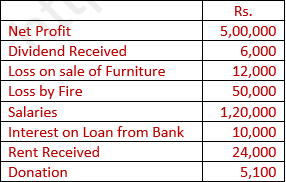Solution 13:

Calculation of Operating Profit:-

Operating Profit = Net Profit − Non-Operating Income + Non-Operating Expenses

Operating Profit = 5,00,000 − 30,000 + 77,100

Operating Profit = Rs 5,47,100

Non-Operating Income = 6,000 + 24,000

Non-Operating Income = 30,000

Non-Operating Expenses = Loss on Sale of Furniture + Loss by Fire + Interest on Loan + Donation

Non-Operating Expenses = 12,000 + 50,000 + 10,000 + 5,100

Non-Operating Expenses = Rs 77,100

Question 14. A merchant has earned a Net Profit of Rs. 57,200 for the year ended 31st March, 2017. Other balances in his Ledger are as under:-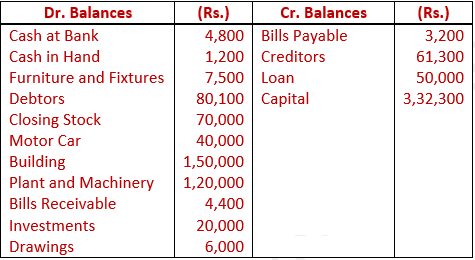Prepare his Balance Sheet as at 31st March, 2017.

Solution 14: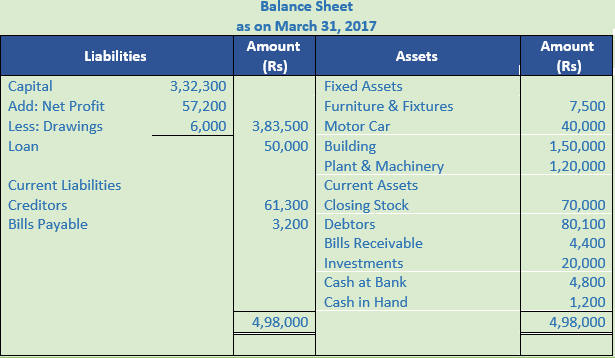Working Note:-

Balance Sheet is prepared with a view to measure true financial position of a business at a particular point of time. It is a method to show the financial position of a business in a systematic and standard form. The financial position of the business can be understood at a glance. The debit and credit balances of related accounts are shown on the assets and liabilities side of the Balance Sheet.

Question 15. The Trial Balance shows the following balances as at 31st March, 2017:-Closing Stock was valued at Rs. 35,000. Prepare Trading and Profit and Loss Account for the year ended 31st March, 2017 and Balance Sheet as at that date.

Solution 15: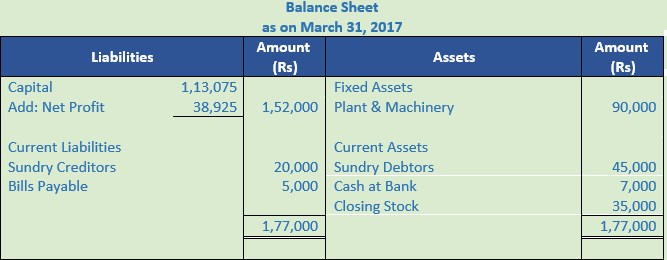Working Note:-

Indirect expenses are those expenses which are incurred and are not directly associated with the purchases of goods or manufacture of goods.

The two examples of indirect expenses are:

1. Office salaries.
2. Warehouse expenses

Question 16. Following is the Trial Balance of Sh. Damodar Parshad as at 31st March, 2016:-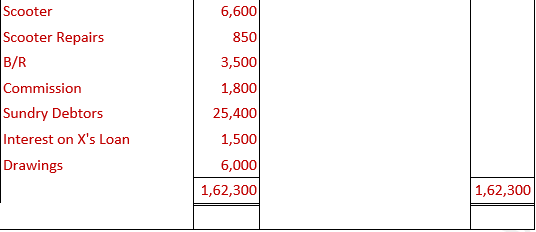Solution 16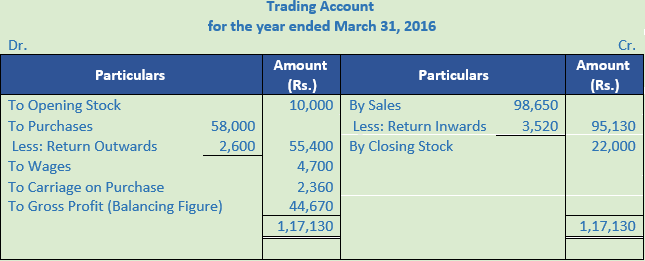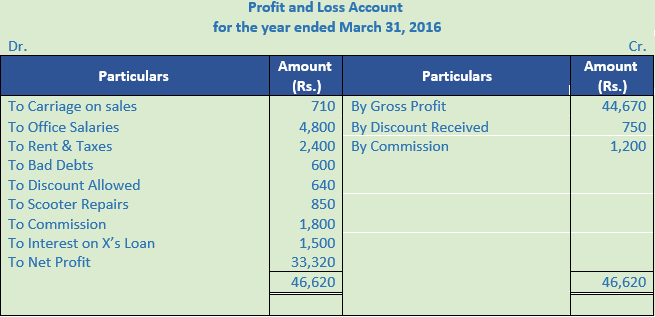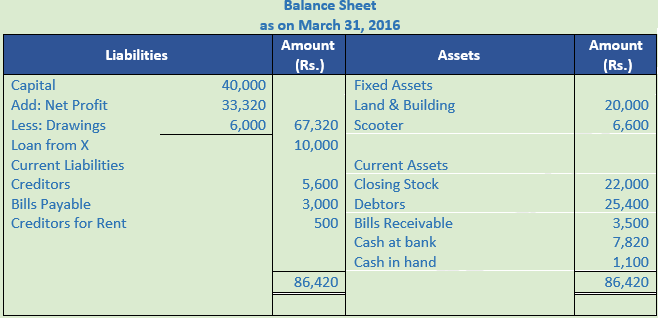Point of Knowledge:-

Current Assets are those assets which are held for resale or for converting into cash. These are the assets which are likely to be realized within a period of one year or during the period of normal operating cycle. A business earns profit by selling these assets but not by keeping them as stock for a longer period. These assets are temporary in nature and may change from time to time. These are sometimes referred to as floating or circulating assets.

Question 17. From the following balances extracted from the books of Sh. Badri Vishal on 31st March, 2017, prepare a Trading Account, P & L A/c and a Balance Sheet. Closing Stock valued on that date was Rs. 15,000.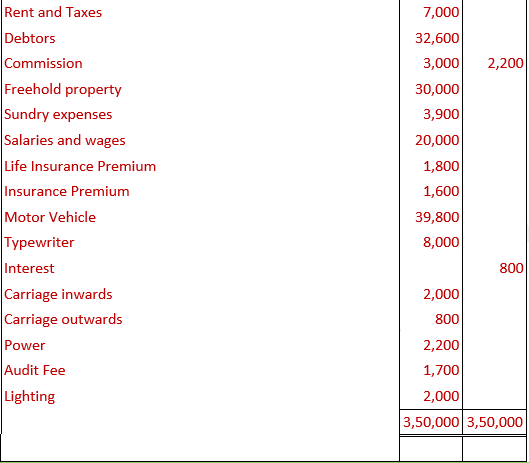Solution 17: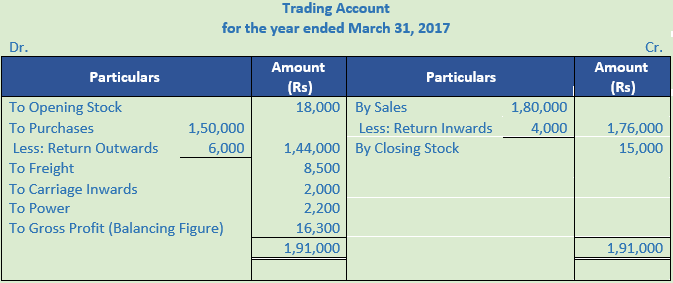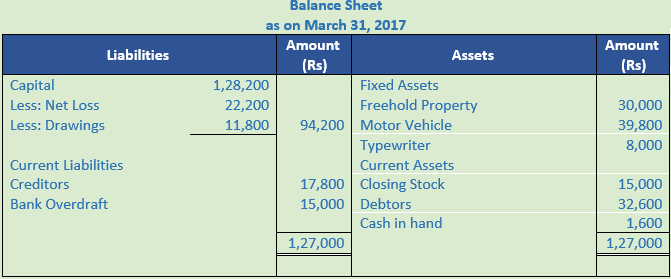Working Note:-

Calculation of Drawings:-

Drawings = Household Expenses + Life Insurance Premium

Drawings = Rs. 10,000 + Rs. 1,800

Drawings = Rs. 11,800

Question 18. From the following balances of the Ledger of Sh. Akhileshwar Singh, prepare Trading and Profit & Loss Account and Balance Sheet :-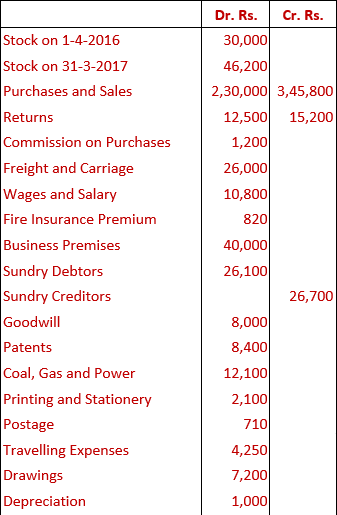Solution 18: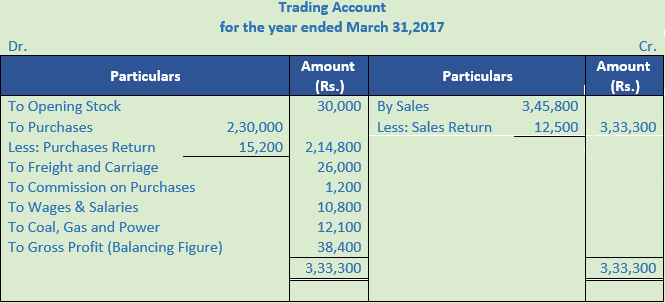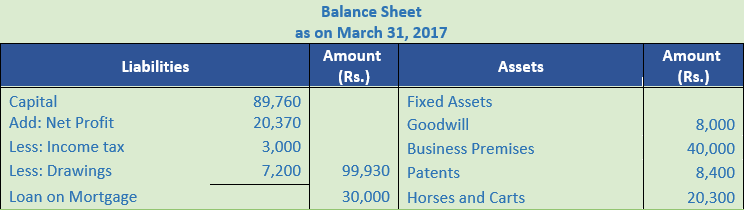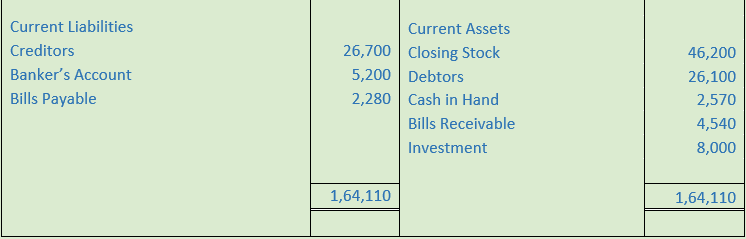Point of Knowledge:-

Current Assets are those assets which are held for resale or for converting into cash. These are the assets which are likely to be realized within a period of one year or during the period of normal operating cycle. A business earns profit by selling these assets but not by keeping them as stock for a longer period. These assets are temporary in nature and may change from time to time. These are sometimes referred to as floating or circulating assets.

Question 19. From the following balances prepare Final Accounts as at 31st March 2017:-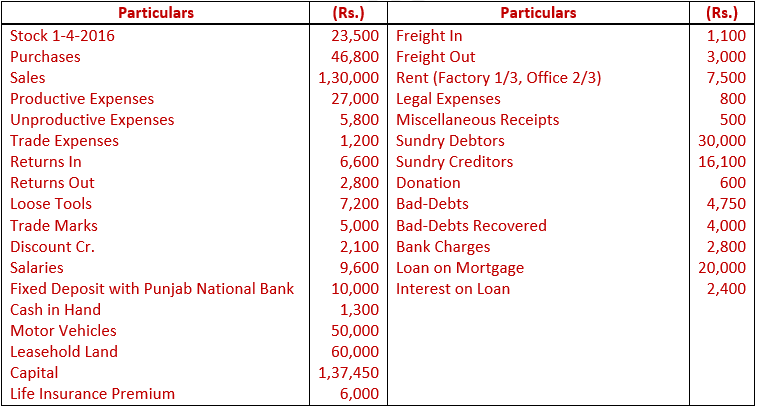Value of Closing Stock was Rs. 36,500 on 31st March, 2017.

Solution 19:Point of Knowledge:-

Direct expenses are those expenses which are incurred on purchases of goods up to the point of bringing them to the place of business. In the case of manufacturing business, they are the expenses incurred to make then ready for sale.

The two examples of direct expenses are:

1. Octroi paid on purchases.
2. Power Expenses

Question 20. Arrange assets in the order of permanence:

Sundry Debtors, Stock, Investment, Land and Building, Cash in Hand, Motor Vehicle, Cash at Bank, Goodwill, Plant and Machinery, Furniture, Loose Tools, Marketable Securities.

Solution 20: Below is the Presentation of assets in the order of Permanence:

1. Goodwill
2. Land and Building
3. Plant and Machinery
4. Motor Vehicle
5. Loose Tools
6. Furniture
7. Investment (Long-term)
8. Stock
9. Sundry Debtors
10. Marketable Securities (Short-term)
11. Cash at Bank
12. Cash in Hand

Point of Knowledge:-

Current Assets are those assets which are held for resale or for converting into cash. These are the assets which are likely to be realized within a period of one year or during the period of normal operating cycle. A business earns profit by selling these assets but not by keeping them as stock for a longer period. These assets are temporary in nature and may change from time to time. These are sometimes referred to as floating or circulating assets.